# Minkowski problem

Does there exist a closed convex hyperplanefor which the Gaussian curvatureis a given function of the unit outward normal? This problem was posed by H. Minkowski , to whom is due a generalized solution of the problem in the sense that it contains no information on the nature of regularity of, even if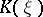is an analytic function. He proved that if a continuous positive function, given on the hypersphere, satisfies the condition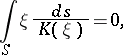(1)

then there exists a closed convex surface, which is moreover unique (up to a parallel translation), for whichis the Gaussian curvature at a point with outward normal.

A regular solution of Minkowski's problem has been given by A.V. Pogorelov in 1971 (see ); he also considered certain questions in geometry and in the theory of differential equations bordering on this problem. Namely, he proved that ifis of class,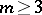, then the surfaceis of class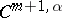,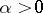, and ifis analytic, thenalso turns out to be analytic.

A natural generalization of Minkowski's problem is the solution of the question of the existence of convex hypersurfaces with given elementary symmetric principal curvature functions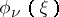of any given order,. In particular, for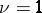this is Christoffel's problem on the recovery of a surface from its mean curvature. A necessary condition for the solvability of this generalized Minkowski problem, analogous to (1), has the form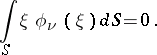However, this condition is not sufficient (A.D. Aleksandrov, 1938, see ). There are examples of sufficient conditions: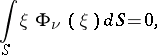where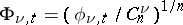,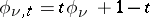,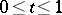. Here the regularity ofis as in the Minkowski problem. Using approximations these results turn out to be valid even for functionswhich are non-negative, symmetric and concave.